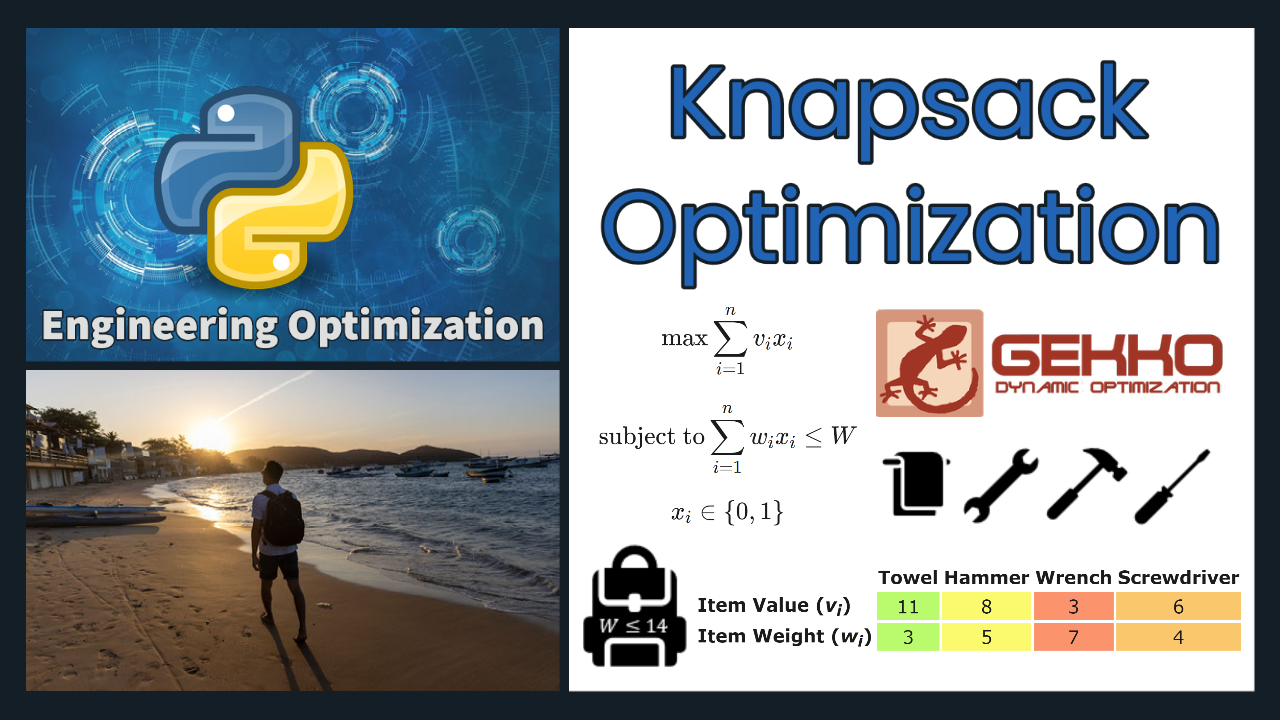## Knapsack Optimization

Objective: Maximize the value of items that can fit into a knapsack without exceeding a maximum weight constraint.There are 4 items available to be placed in a knapsack: a towel, hammer, wrench, and screwdriver. The value and weight of the items are listed in the table below.Towel Hammer Wrench Screwdriver Item Value (vi) 11 8 3 6 Item Weight (wi) 3 5 7 4

The purpose of the knapsack problem is to select which items to fit into the bag without exceeding a weight limit of what can be carried. We solve the problem with an integer programming solver (APOPT) by setting up each item as a binary variable (0 or 1). A zero (0) is a decision to not place the item in the knapsack while a one (1) is a decision to include it. More generally, suppose there are n items with values v_1, \ldots, v_n and weights w_1, \ldots, w_n with a total weight limit of the sack W=14. The decision variables are x_1, \ldots, x_n that can be 0 or 1. The following optimization formulation represents this problem as an integer program:

$$\max \sum _{i=1}^{n} v_{i} x_{i}$$

$$\textrm{subject to} \sum _{i=1}^{n} w_{i} x_{i}\leq W$$

$$x_{i}\in \{0,1\}$$

An alternative to using optimization is a greedy algorithm where the items are successively selected based on a metric such as the highest value to weight ratio. This is done until the weight limit is exceeded. While this approach is computationally fast and intuitive, it may give suboptimal results because a constraint limit (weight) is not reached. The greedy algorithm is an example of a heuristic (rule-based) approach that is often specific to the application. Heuristics may be valuable to initialize the optimization solution or identify at least one feasible solution that can be improved with optimization.

#### Python GEKKO Solution

from gekko import GEKKO

y = ['towel','hammer','wrench','screwdriver']
v = [11,8,3,6]
w = [3,5,7,4]
items = len(y)

# Create model
m = GEKKO()

# Variables
x = m.Array(m.Var,len(y),lb=0,ub=1,integer=True)

# Objective
m.Maximize(m.sum([v[i]*x[i] for i in range(items)]))

# Constraint
limit = 14
m.Equation(m.sum([w[i]*x[i] for i in range(items)]) <= limit)

# Optimize with APOPT
m.options.SOLVER = 1

m.solve()

# Print the value of the variables at the optimum
for i in range(items):
print("%s = %f" % (y[i], x[i].value))

# Print the value of the objective
print("Objective = %f" % (-m.options.objfcnval))